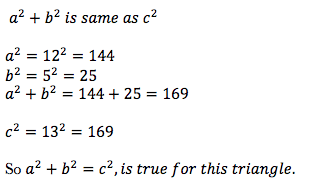# Practice 2 – Application of Pythagorean Theorem

Questions :

Calculate the hypotenuse of a right triangle with sides 7in and 24in long.

If the hypotenuse of a right triangle is 8 inches long and one of the sides measures 2 inches, how long is the other side?

If one side of an equilateral triangle measures 6 cm, then find the measure of its height.

The sides of a triangle measure 13 in, 12 in and 5 in. Prove that it’s a right angle triangle.

Explanations :

Step 1 : Draw a diagram.Step 2: Use the Pythagorean theorem to find the answer.Step 1 : Assign letters to the sides.

Side a=2 in

Side b= ?

c=8 in

Step 2: Use the Pythagorean theorem to find the answer.Step 1 : Draw a diagram. All 3 sides of an equilateral triangle are the same.Step 2: Use the Pythagorean theorem to find the answer.Step 1 :  Think – what is the property of the sides of a right triangle?

That means square of the longest side is the sum of the squares of the other 2 sides.

Lets’ give some name to the sides:

a=12 in

b=5 in

c=13 in

Step 2: Now prove that